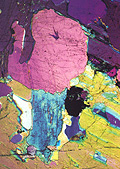### Cluster State Quantum Computing

Cluster states form an alternative approach to quantum computing. The heart of this architecture is to create a large entangled state as a resource. The computation then proceeds as a series of (parallel) single-qubit measurements. Since all the entanglement is produced off-line, this is a particularly powerful approach for single-photon quantum computing.

Before we introduce cluster states, we must cast arbitrary single-qubit rotations into arbitrary rotations around the $Z$ axis and Hadamard operations $H$. Using $H^2 = I$ and $X = HZH$, any arbitrary rotation $R$ can be decomposed into three Euler angles $\alpha$, $\beta$, and $\gamma$: $$R(\theta) = Z(\gamma)\, X(\beta)\, Z(\alpha) = H\, H Z(\gamma)\, H Z(\beta)\, H Z(\alpha)$$ where $Z(\alpha) = \exp(i \alpha Z/2)$ and $X(\beta) = \exp(i \beta X/2)$. In circuit language, this becomes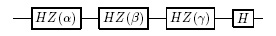and I will now show how $HZ(\alpha)$ can be implemented via a single-qubit measurement.

Consider the following circuit diagram of single-qubit teleportation:The measurement is in the computational basis, and the outcome $m$ takes the value 0 or 1. Depending on this value, we apply a Pauli $Z$ operation to the teleported qubit.

The next step is to translate the CNOT gate into the CZ gate. This procedure incurs two Hadamard gates, which are absorbed into the state of the ancilla ($|0\rangle$ to $|+\rangle$) and the teleported qubit.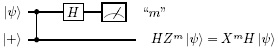A single-qubit rotation around the $Z$ axis to the input qubit $|\psi\rangle$ can be written as:The rotation around the $z$-axis commutes with the CZ operation (they are both diagonal in the computational basis), and we can write: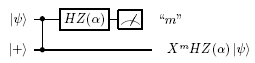We can reinterpret this diagram as an entangled state $|\psi\rangle = CZ |\psi,+\rangle$, followed by a single-qubit measurement on the first qubit that performs the single-qubit gate $HZ(\alpha)$ on the state $|\psi\rangle$. The precise measurement basis $A(\alpha)$ is determined by $$\langle\psi| Z(-\alpha) H Z H Z(\alpha) |\psi\rangle = \langle\psi| Z(-\alpha) X Z(\alpha) |\psi\rangle = \langle\psi| A(\alpha) |\psi\rangle$$ This corresponds to a measurement along an axis in the equatorial plane of the Bloch sphere.

In order to implement an arbitrary rotation $R(\theta)$, this procedure must be concatenated three timeswith $$|\psi_{\rm out}\rangle = (X^m H Z(\gamma)) (X^l H Z(\beta)) (X^k H Z(\alpha)) |\psi\rangle$$ However, the operators $X^k$, $X^l$, and $X^m$ depend on the measurement outcomes, and we should try to get rid of them by commuting them through the Pauli gates and Hadamards. We can again use the relations $ZX = -XZ$ to show that $$Z(\beta) X = \sum_{n=0}^{\infty} \left( \frac{i\beta}{2} \right)^n \frac{Z^n X}{n!} = \sum_{n=0}^{\infty} \left( \frac{-i\beta}{2} \right)^n \frac{XZ^n}{n!} = XZ(-\beta)$$ This therefore gives rise to an adjustment of the measurement bases depending on the previous measurement outcomes, and it results in a definite temporal direction in the computation. Hence the name "one-way model" of quantum computing. (Remember that all the elements in the traditional circuit model are unitary operators, and therefore reversible.)

Now that we have constructed arbitrary single-qubit operations, we do not need to start our circuit with the input state $|\psi\rangle$, but we can start with another $|+\rangle$ state and implement the first single-qubit rotation to obtain the required input state $|\psi\rangle$. We can then write the evolution of a single qubit graphically as a string of ancilla qubits in state $|+\rangle$ (circles), connected via CZ operations (edges):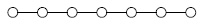Multi-qubit evolution is then represented as a collection of such strings. The strings can be bridged vertically by edges, which in turn induce two-qubit operations: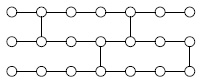When all the nearest-neighbour connections are established, and the qubits form an entangled grid, any quantum circuit can be realized if the cluster state is large enough. Such a state is called a universal cluster state.

To show that a vertical bridge induces a CZ gate, consider the following sequence of measurements and entangling operations:We start with two rows of three qubits without any entanglement (a). The two rows will form the two qubits we wish to apply the CZ gate to. We then entangle qubit 1 with qubit 2 in each row (b) and measure qubits 1 (c). After the first two measurements, the states of qubits 1 are transferred to qubits 2. We can then apply the CZ gate to the two qubits, as well as the two CZ gates that connect qubits 2 with qubits 3 (d). Finally, we measure qubits 2 and transfer the quantum information to the output qubits 3 (e). The point here is that we can apply the CZ gate as if we are using it in the circuit model. However, because the measurements and the cz operations in this sequence commute, we could have created all the entanglement at the start. Hence the vertical CZ gates are suitable as two-qubit gates in cluster state quantum computing.

An often heard objection to cluster state quantum computing is that it seems to be very wasteful with entanglement. Instead of having to create entanglement for every two-qubit gate in an $N$-qubit computation, we seem to need at least $N$ entangling operations for every clock cycle! However, this is far too pessimistic. There is an enormous redundancy in a cluster state that is translated straight from the circuit model, as we did above. First of all, we can perform all single-qubit operations in the Clifford group before the computation starts: These operations also correspond to measurements, but their outcome does not affect any subsequent choice of measurement basis and can therefore be carried out at any stage. Also, these measurements will turn cluster states into smaller cluster states. As a result, we can calculate the effect of most Clifford operations and create a minimal cluster state that is in fact much smaller than what we found in the translation from the circuit model. Since most of the error correction in the quantum computation involves Clifford operations, this is a huge saving. Secondly, we do not have to create the complete cluster state for the computation all at once. We can create a cluster with a relatively shallow depth, and keep adding qubits to the right as we measure qubits on the left. That way, we make our cluster "just in time". The fusion gates can be used to create these cluster states with a moderate overhead per qubit, as I will show in the next section.